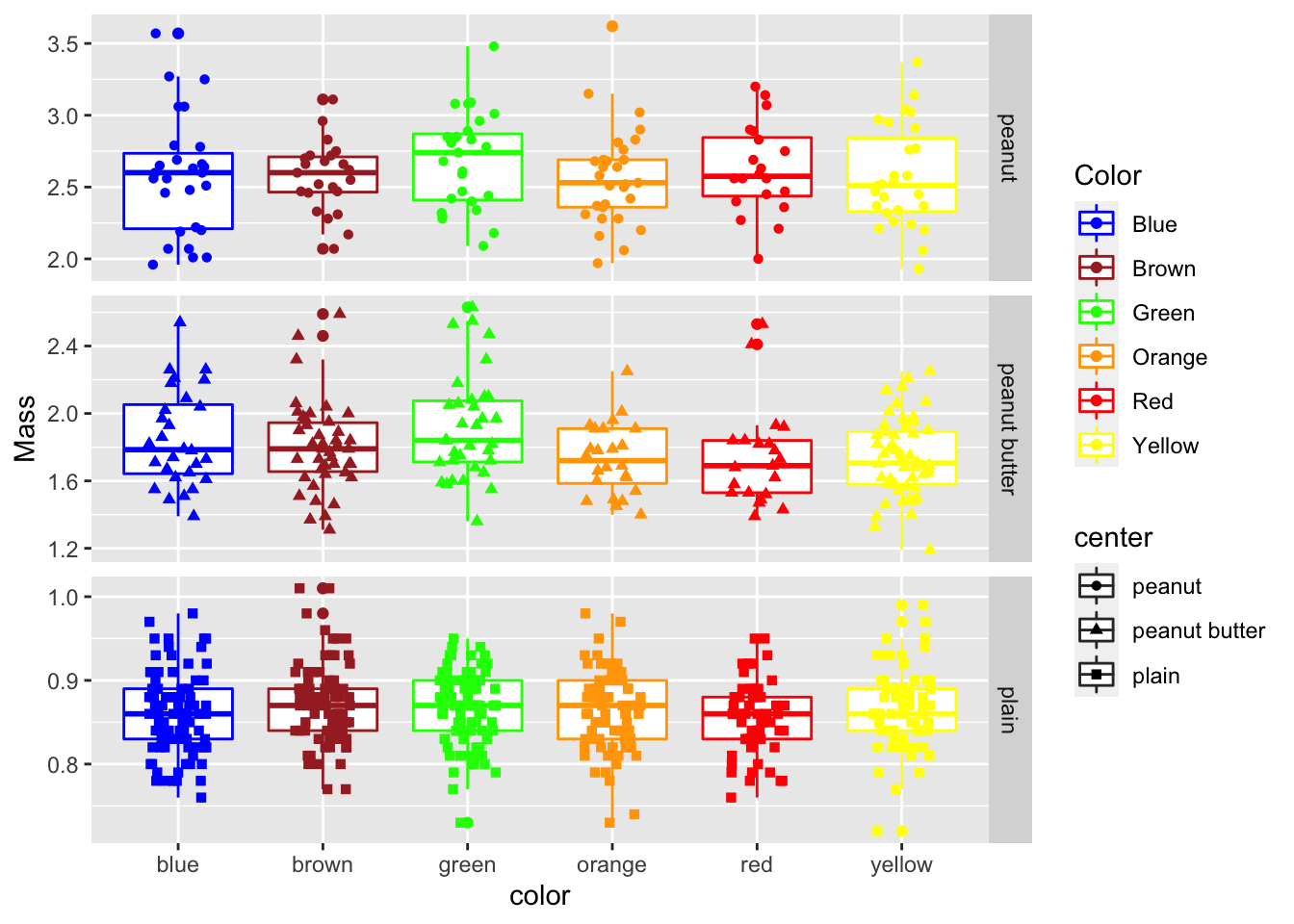``````# install.packages("devtools")
# devtools::install_github("thomasp85/patchwork")

# load the libraries each time you restart R
library("tidyverse") # dplyr and piping and ggplot etc
library("lubridate") # dates and times
library("scales") # scales on ggplot ases
library("skimr") # quick summary stats
library("janitor") # clean up excel imports
library("patchwork") # multipanel graphs``````

``````# So now we have seen how to look at the data
# What if we wanted to modify the data in terms of columns or rows

# Making graphs this way can get a bit cumbersome as you might imagine.
# This is because the data is in what we call wide format
# The long format is the format often used for Anovas and other stats
# We will go over how to do this later but for now lets just look at the file

``````##
## ── Column specification ────────────────────────────────────────────────────────
## cols(
##   center = col_character(),
##   color = col_character(),
##   diameter = col_double(),
##   mass = col_double()
## )``````
``````# Plotting long format data ------
# notice that it plots all the data and is sort of a mess...
# there are no groupings of cladocerans or copepods

ggplot(mm.df, aes(color, mass)) + # sometimes necessary is , group = group
geom_point()``````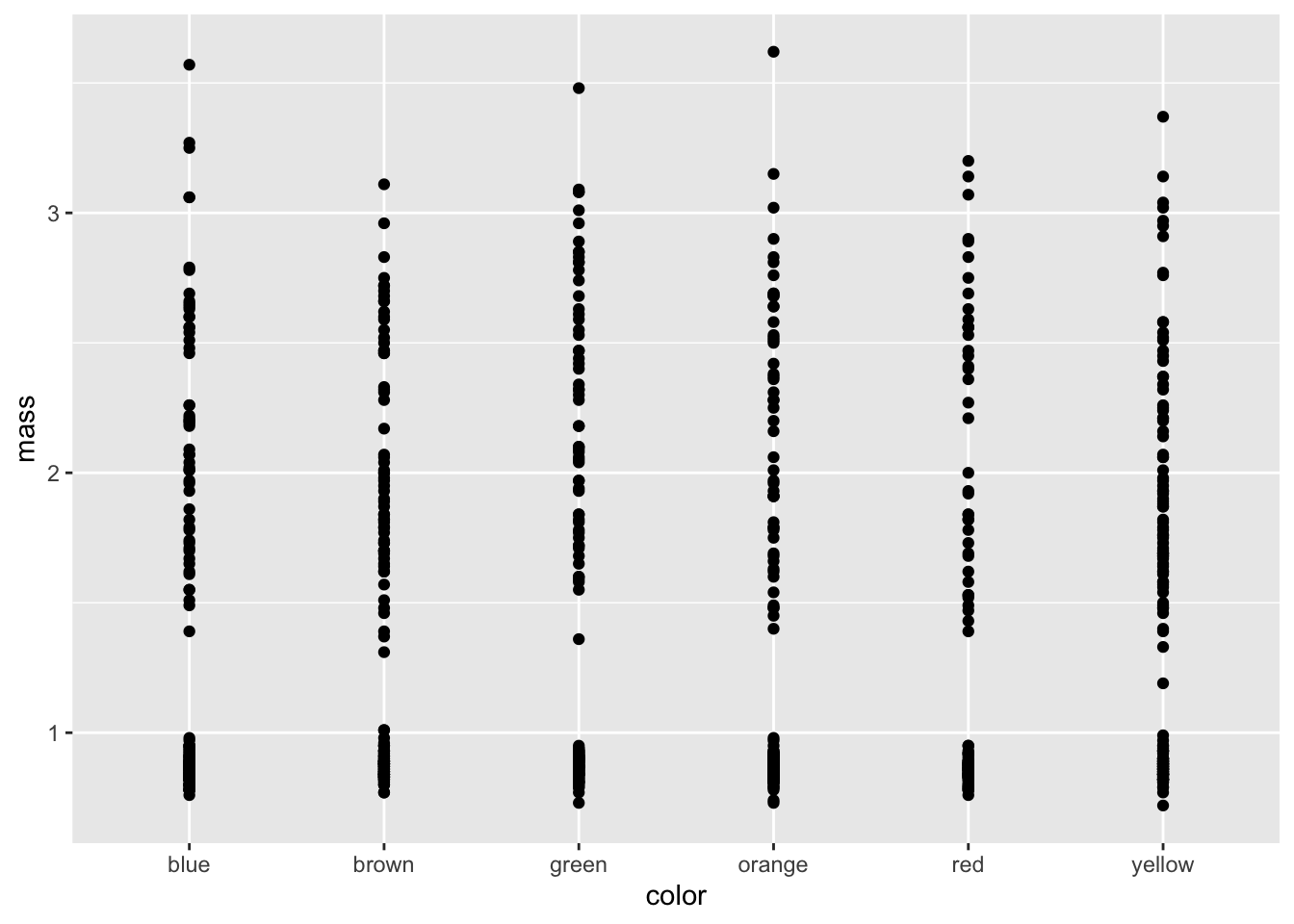``````# Mapping a color to data groups ----
# If you add ", color=group" inside of the aes statement it will map a color to
# each group and it is sometimes necessary to add ", group = group"
ggplot(mm.df, aes(color, mass, color=color)) +
geom_point()``````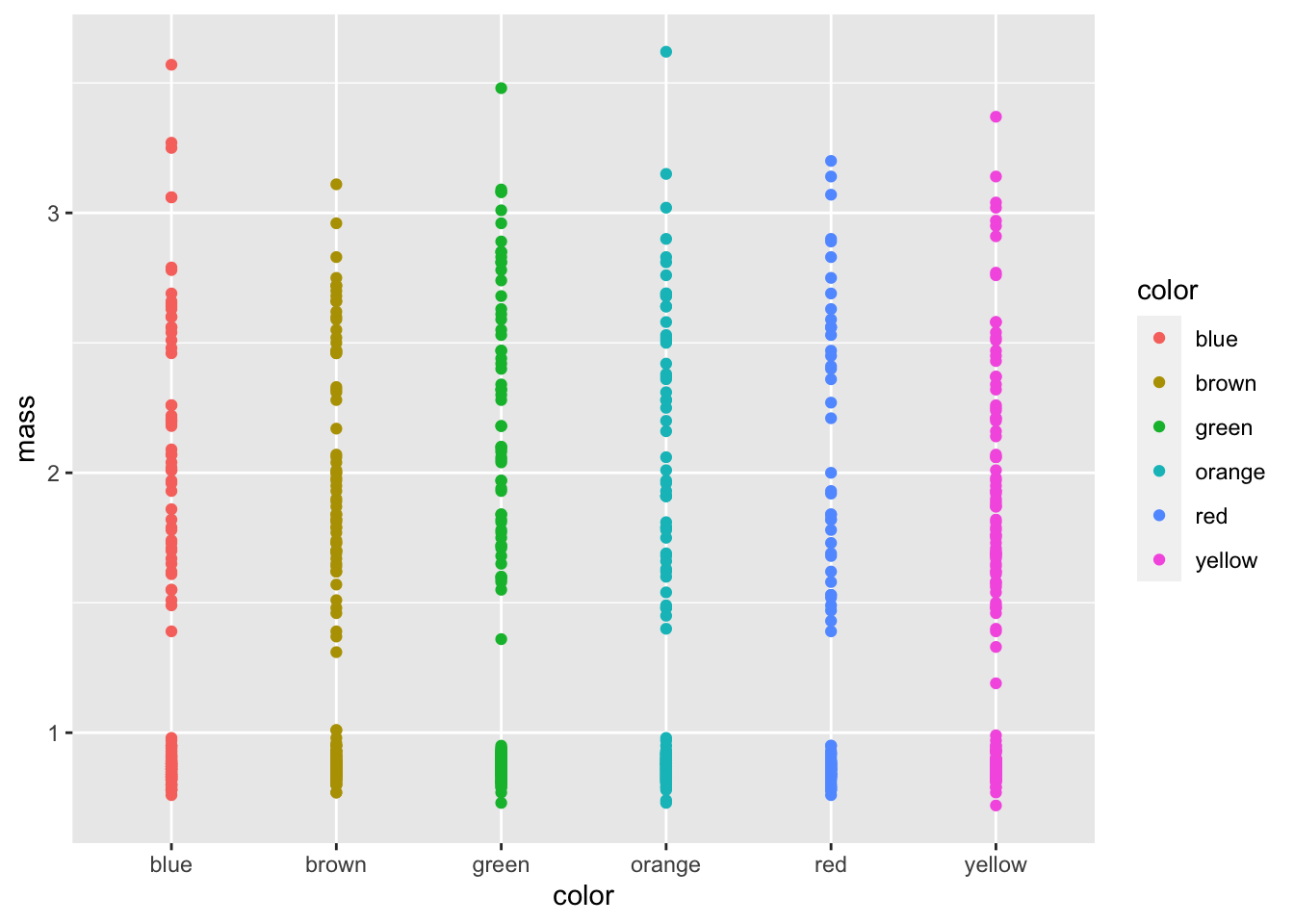We know shape is in there so we should add that

``````ggplot(mm.df, aes(color, mass, color=color, shape = center)) +
geom_point()``````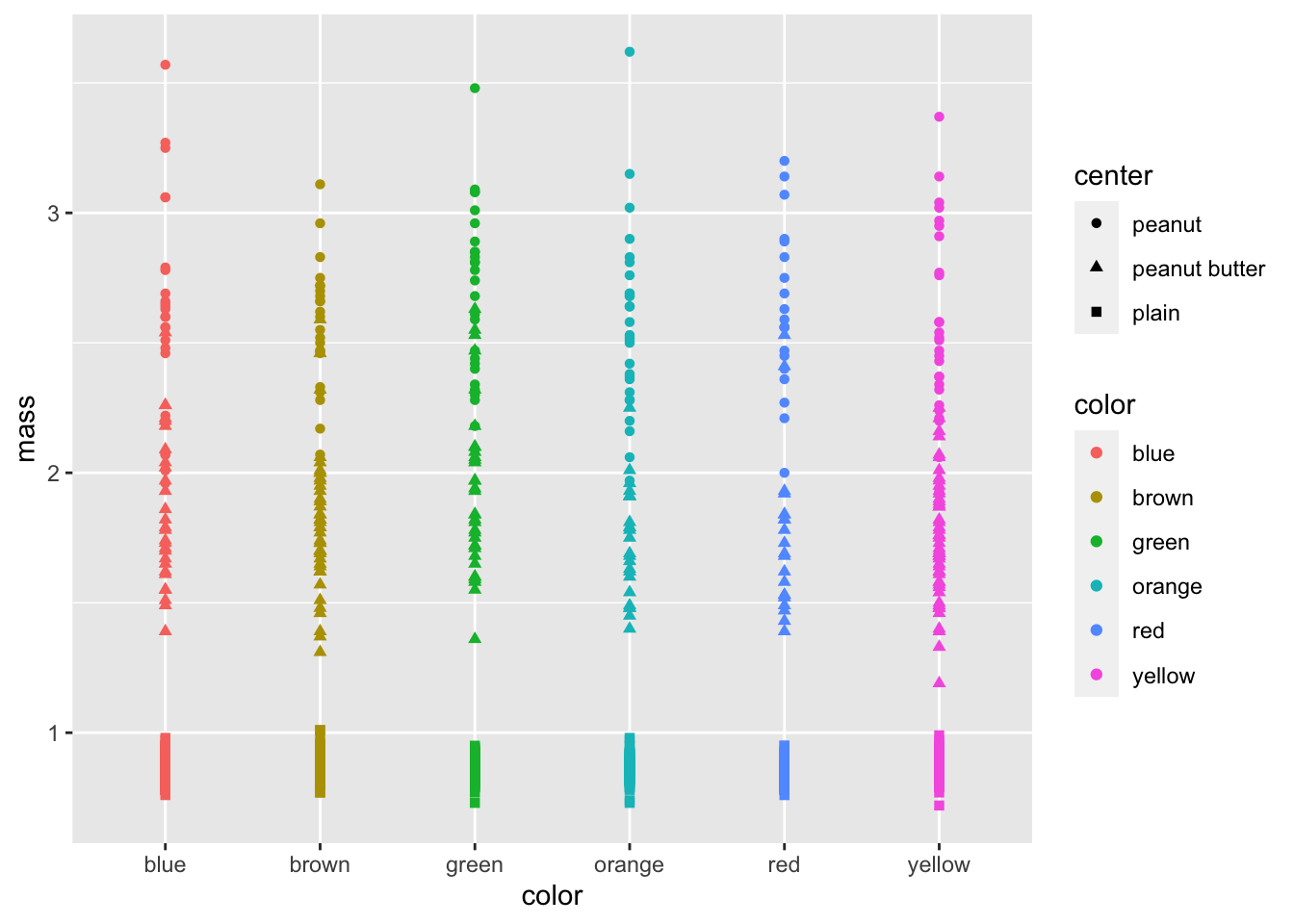# Dodging points Now lest try to dodge the points

``````ggplot(mm.df, aes(color, mass, color=color, shape = center)) +
geom_point(position= position_jitterdodge(jitter.width = 0.4))``````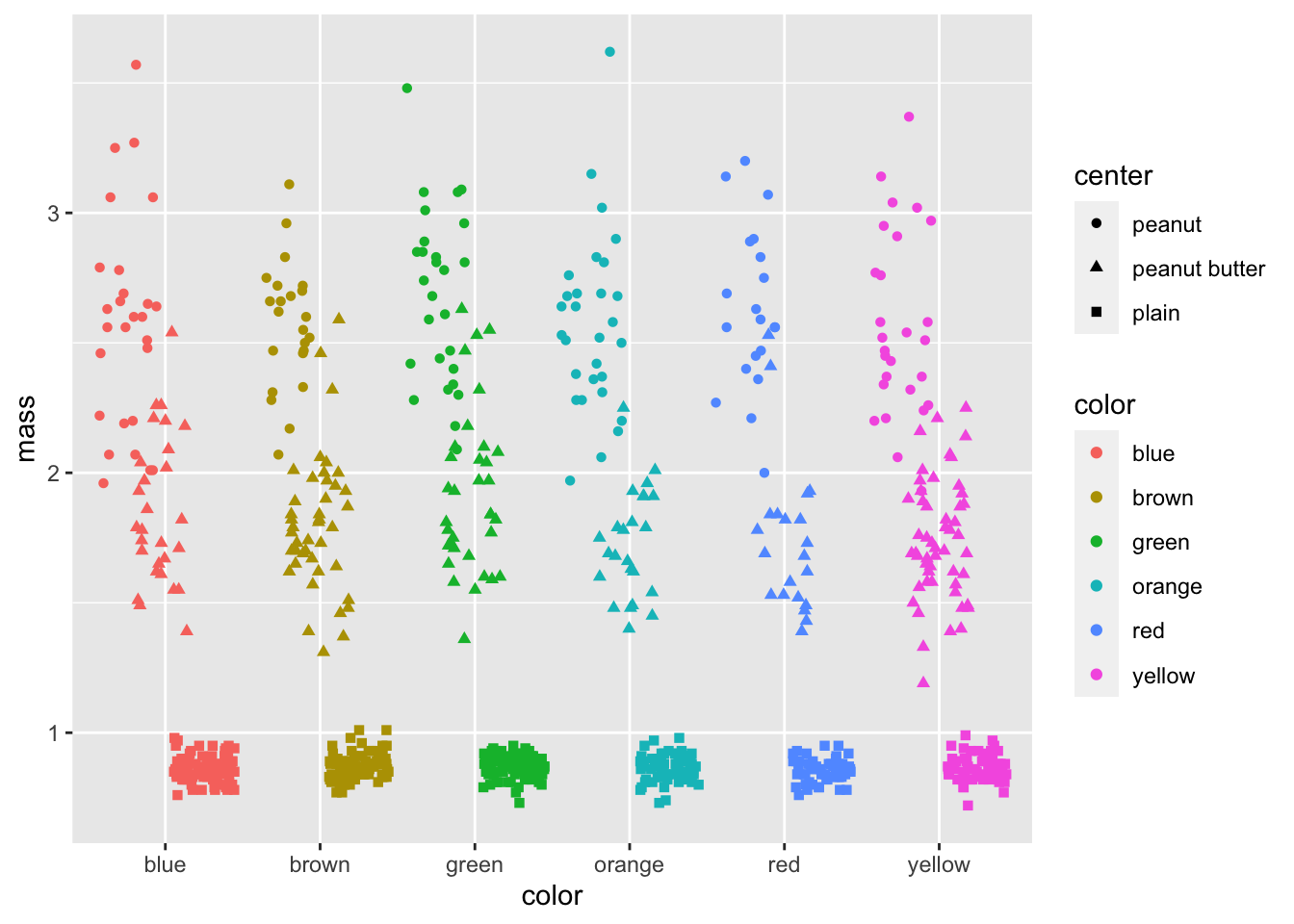``````# Now lets look at some statistical plot

ggplot(mm.df, aes(color, mass, color=color, shape = center)) +
geom_point(position= position_jitterdodge(jitter.width = 0.4)) ``````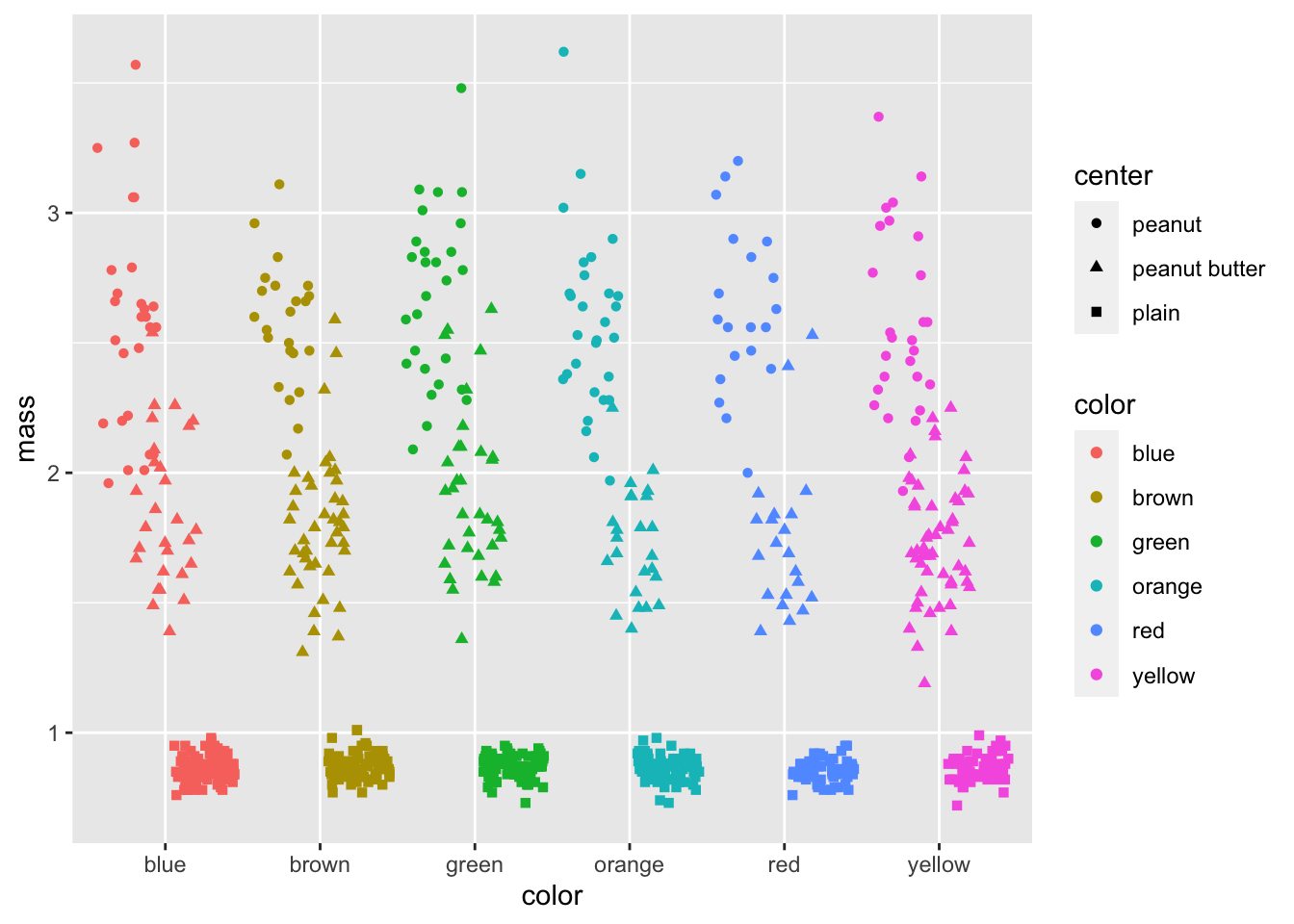``````# Final publication quality graph-----
# now we can add axes labels and custom colors
ggplot(mm.df, aes(color, mass, color=color, shape = center)) +
geom_boxplot() +
geom_point(position= position_jitterdodge(jitter.width = 0.4)) +
labs(x = "color", y = "Mass") +
scale_color_manual(name = "Color",
values = c("blue", "brown", "green", "orange", "red", "yellow"),
labels = c("Blue", "Brown", "Green", "Orange", "Red", "Yellow"))``````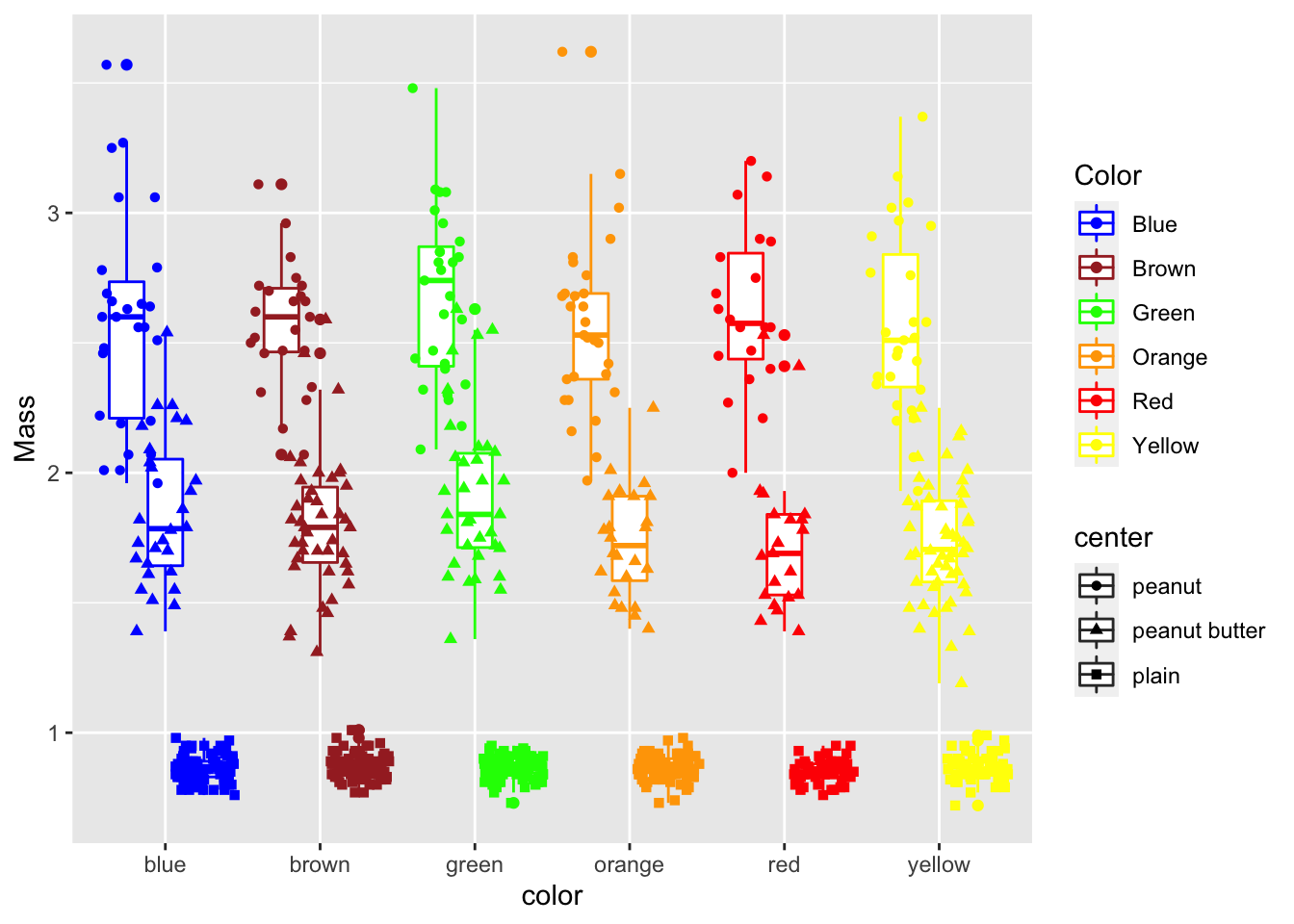# Facetting graphs
what if we wanted to break up this graph

``````ggplot(mm.df, aes(color, mass, color=color, shape = center)) +
geom_boxplot() +
geom_point(position= position_jitterdodge(jitter.width = 0.4)) +
labs(x = "color", y = "Mass") +
scale_color_manual(name = "Color",
values = c("blue", "brown", "green", "orange", "red", "yellow"),
labels = c("Blue", "Brown", "Green", "Orange", "Red", "Yellow"))+
facet_wrap(~center)``````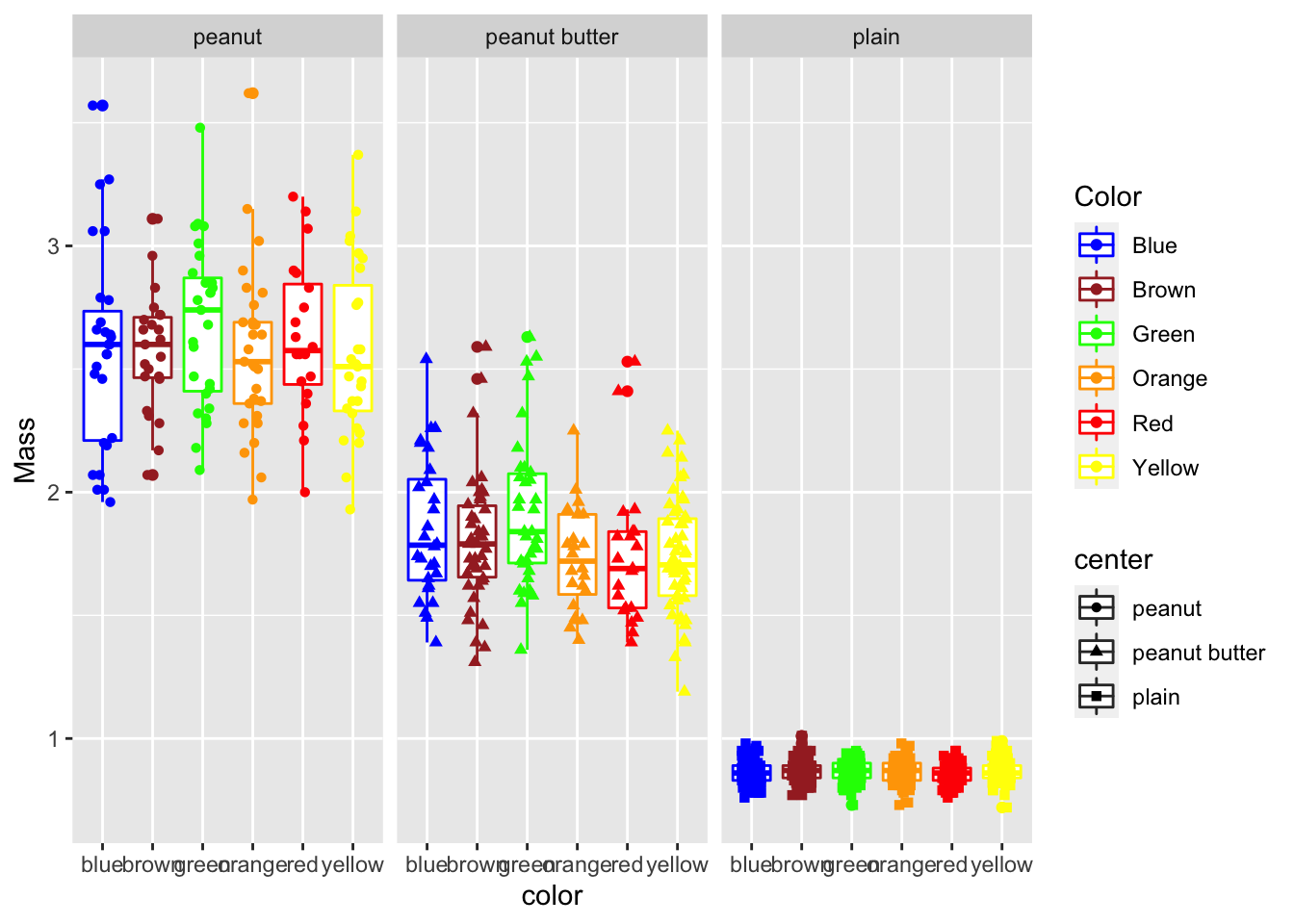``````# Facet_grid -----
# lets you make a grid of one or two variables in a grid

ggplot(mm.df, aes(color, mass, color=color, shape = center)) +
geom_boxplot() +
geom_point(position= position_jitterdodge(jitter.width = 0.4)) +
labs(x = "color", y = "Mass") +
scale_color_manual(name = "Color",
values = c("blue", "brown", "green", "orange", "red", "yellow"),
labels = c("Blue", "Brown", "Green", "Orange", "Red", "Yellow"))+
facet_grid(center~., scales="free_y")``````